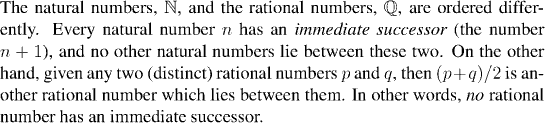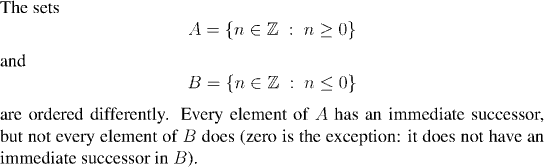Analysis WebNotes
arrow_back arrow_forward

# Class Contents

## Properties of Ordered Sets

As we saw above, one of the axioms of the real numbers is going to be that the real numbers are an ordered field. The remaining axiom only involves the order structure of the real numbers, so in this section we will concentrate on linearly ordered sets in general.

We should start by looking at a couple of examples to see how different sets can be distinguished by properties that have only to do with the ordering.

Example:Example:Example:

The property that characterizes the real numbers has got something of the flavor of this last example, but is more complicated. In order to describe it we need to have a number of definitions, working towards the definition of supremum and the least upper bound property

## Bounded SetsExample:( Prove this using the axioms for an ordered field.) But note that 2, 3.9, and, for that matter, 57,000,000, or, in fact, any other rational number bigger than 1, is also an upper bound for S.

Note that none of these upper bounds belong to S. In fact, S doesn't have an upper bound that belongs to it. (Remark) . If such an element exists it is called the greatest or largest element of the set.

• In general a set which is bounded above can have many upper bounds.

Let's concentrate on this issue of a set having many upper bounds. What makes one upper bound "better" than another? If some bounds are better than others, what would be the best possible upper bound? How could we recognize it, if we had a best upper bound?